# Deep Learning Models with Tensorflow 2.0Interpretability of Deep Learning Models with Tensorflow 2.0

This article dives into the tf-explain](https://www.github.com/sicara/tf-explain) library. It provides explanations on interpretability methods, such as Grad CAM, with Tensorflow 2.0.

Did you ever wait long hours for training that turns out unsuccessful? I have, and not just once. So I began looking into tools that could help me anticipate and debug neural networks that come out of training.

• A visual logging to check pipeline integrity

• Interpretability methods to have insights on the inside of neural networks

All the methods presented in this article are implemented intf-explain, a library built for interpretability with TensorFlow 2.0. Check out the introduction!

Let’s look now at those implementations.

## Look at what comes in the network

The first cause for failed trainings is simply not giving the network what you want him to have. Visualizing the inputs is crucial, and for that, I use the VisualLogging library. Dropping sample images as logs at different key moments of your pipeline (loading, resizing, data augmentation) can help you catch eventual undesired effects.

``````import logging

import cv2
import numpy as np
import vlogging

logger = logging.getLogger("demo")
fh = logging.FileHandler('test.html', mode="w")

logger.setLevel(logging.DEBUG)

logger.info(vlogging.VisualRecord('Sample Image', image, {'footnote': 'sample image'}, fmt='png'))
``````

visual_logging.py

Logs are directly dropped into an HTML page, which you can scroll and inspect.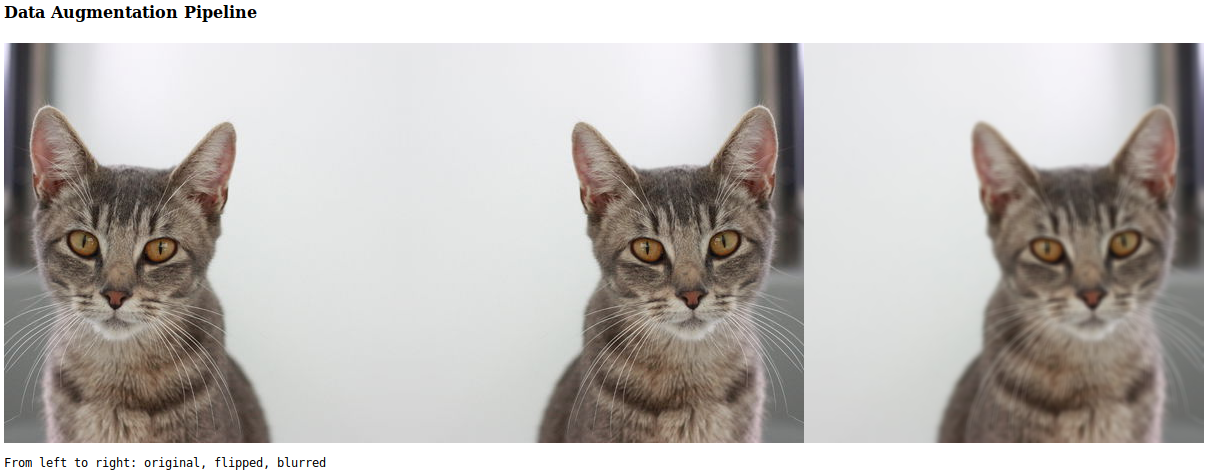Example of a possible Data Augmentation Pipeline

## Monitor Convolutional Kernels

Let’s now dive into interpretability methods. Key components of convolutional nets are kernels. All that matters is what the kernel is learning, how its output contributes to the final classification.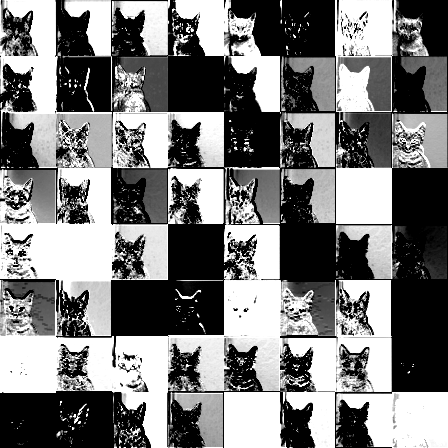Outputs of ResNet50’s activation_1 layer for a sample cat

### Intermediate Layers Visualization

A first step is to simply visualize what comes out of the activation layers. Does the output still look relevant? Or does it look like random noise? By examining how the image transits through the network, you can validate that it focuses on the right regions.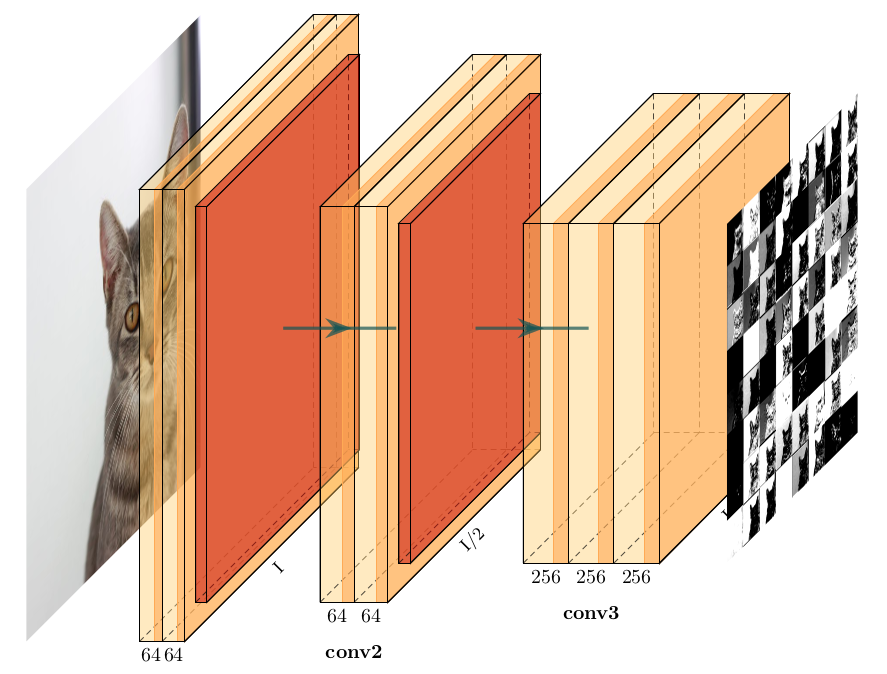Subgraph of VGG16 to observe activations

Subgraph of VGG16 to observe activations

Extracting the output of an intermediate layer with Tensorflow is fairly easy. You start from your whole model and extract a subpart of the graph. The code below shows how to obtain the outputs of the `activation_1` layer from a Resnet50 model.

``````import numpy as np
import tensorflow as tf

layers_name = ['activation_1']
IMAGE_PATH = './cat.jpg'

# Model to examine
model = tf.keras.applications.resnet50.ResNet50(weights='imagenet', include_top=True)

# Image to pass as input
img = tf.keras.preprocessing.image.img_to_array(img)

# Get the outputs of layers we want to inspect
outputs = [
layer.output for layer in model.layers
if layer.name in layers_name
]

# Create a connection between the input and those target outputs
activations_model = tf.keras.models.Model(model.inputs, outputs=outputs)

# Get their outputs
activations_1 = activations_model.predict(np.array([img]))``````

activations_visualization.py

### Kernel Inspection

Seeing what is coming out of a layer is great, but what if we could understand what makes a kernel activate?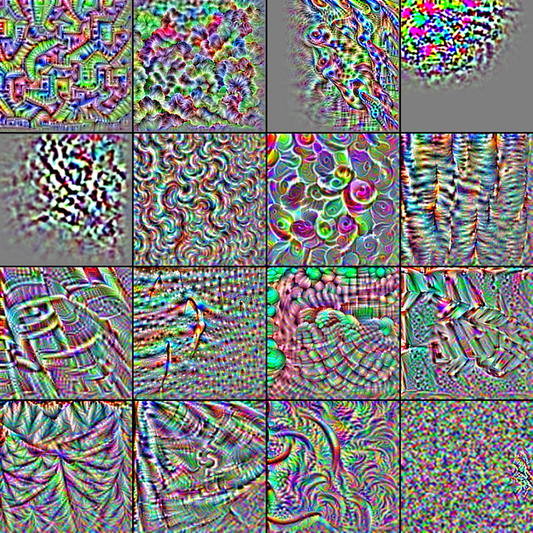Visualization of VGG Filters

The idea behind this visualization is to generate an input to the network that maximizes the reaction of a given kernel filter. Therefore, we create a sub-model which stops at the target layer. The loss function we seek to maximize is the mean of this activation layer’s output. The starting point is taking some random noise as input. Then, we backpropagate the gradients to perform gradient ascent on the noise. Iteratively, we build an input that makes the filter’s reaction stronger and stronger.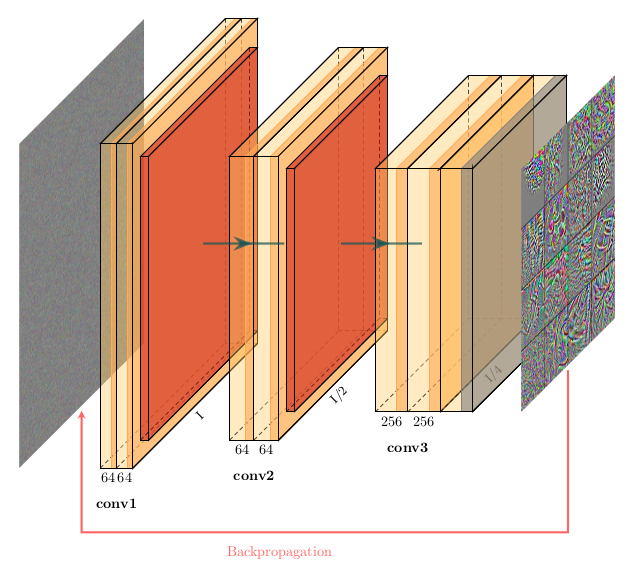Gradient Ascent on Input to Visualize Kernels

With Tensorflow, the implementation of this method is only 4 steps:

• perform the initial subgraph creation (same as before)

• use the `GradientTape` object to capture the gradients on the input

• get the gradients with `tape.gradient`

• perform the gradient ascent with `assign_add` on the initial variable.

``````import numpy as np
import tensorflow as tf

# Layer name to inspect
layer_name = 'block3_conv1'

epochs = 100
step_size = 1.
filter_index = 0

# Create a connection between the input and the target layer
model = tf.keras.applications.vgg16.VGG16(weights='imagenet', include_top=True)
submodel = tf.keras.models.Model([model.inputs], [model.get_layer(layer_name).output])

# Initiate random noise
input_img_data = np.random.random((1, 224, 224, 3))
input_img_data = (input_img_data - 0.5) * 20 + 128.

# Cast random noise from np.float64 to tf.float32 Variable
input_img_data = tf.Variable(tf.cast(input_img_data, tf.float32))

for _ in range(epochs):
outputs = submodel(input_img_data)
loss_value = tf.reduce_mean(outputs[:, :, :, filter_index])
``````

kernel_visualization.py

The example here is minimal to keep the code simple. Many techniques exist to improve those kernel visualizations (regularization, upscaling). If you are interested in this subject, I strongly encourage you to read this blog post on Feature Visualization.

## What Makes the Neural Network’s Decision

Visualizing the kernels and the intermediate layers can help detect weird behaviors. However, it does not give any insights on why a neural network makes a specific decision. The next few methods are ways to visualize what part of the input is influencing the output value.

### Occlusion Sensitivity

The idea behind Occlusion Sensitivity is to hide parts of the image and see the impact on the neural network’s decision for a specific class.

On the animation below, we run a blue patch over a cat image and extract the confidence at each step. When the patch goes over the cat, confidence drops, so we can identify the region behind the patch as hot. When the patch does not occlude the cat, the confidence stays even or eventually goes up. This happens because we potentially hide elements that degrade the performance.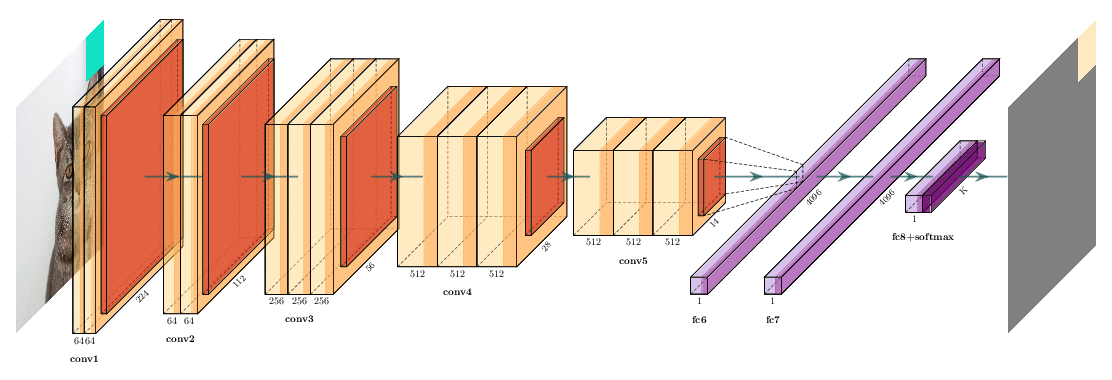Heatmap generation process for class Cat

The heatmap generated carries the information “Does this part of the image helps to improve confidence”. Here, the resolution is pretty poor. You can improve it by varying the patch size to capture influences from micro to macro zones of the image.

The process to generate the heatmap is decomposed simply :

• Create a batch of images with patches applied

• Run predictions

• Save confidence for the target class

• Regroup confidences in the resulting map

``````import numpy as np
import tensorflow as tf

# Create function to apply a grey patch on an image
def apply_grey_patch(image, top_left_x, top_left_y, patch_size):
patched_image = np.array(image, copy=True)
patched_image[top_left_y:top_left_y + patch_size, top_left_x:top_left_x + patch_size, :] = 127.5

return patched_image

IMAGE_PATH = './cat.jpg'
img = tf.keras.preprocessing.image.img_to_array(img)

# Instantiate model
model = tf.keras.applications.resnet50.ResNet50(weights='imagenet', include_top=True)

CAT_CLASS_INDEX = 281  # Imagenet tabby cat class index
PATCH_SIZE = 40

sensitivity_map = np.zeros((img.shape, img.shape))

# Iterate the patch over the image
for top_left_x in range(0, img.shape, PATCH_SIZE):
for top_left_y in range(0, img.shape, PATCH_SIZE):
patched_image = apply_grey_patch(img, top_left_x, top_left_y, PATCH_SIZE)
predicted_classes = model.predict(np.array([patched_image]))
confidence = predicted_classes[CAT_CLASS_INDEX]

# Save confidence for this specific patched image in map
sensitivity_map[
top_left_y:top_left_y + PATCH_SIZE,
top_left_x:top_left_x + PATCH_SIZE,
] = confidence

``````

Occlusion Sensitivity Implementation

Note: This code translates the algorithm logic, but should be optimized by first generating all the patched images and then running the predictions in batches.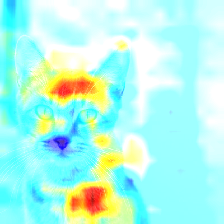### Class Activation Maps

Another type of methods uses directly the gradients to determine the relevant zones. Class Activations Maps (CAM) and more specifically Grad-CAM methods (which is implemented below) check the importance of output filters(see section `Intermediate Layers Visualization` above) towards the final decision.

Given those convolutional filters (of shape `WxHxN`), we compute the gradients towards the class score (same shape `WxHxN`). To establish the importance of each filter in the decision, we take the average of its weights (with shape `1x1xN)` as a judge, and multiply each map by its corresponding weights. Then, we sum up all those ponderated maps into a final heatmap. If an activation map has been lightened up during forward pass, and if its gradients are large, it means the region which is activated has a large impact on the decision.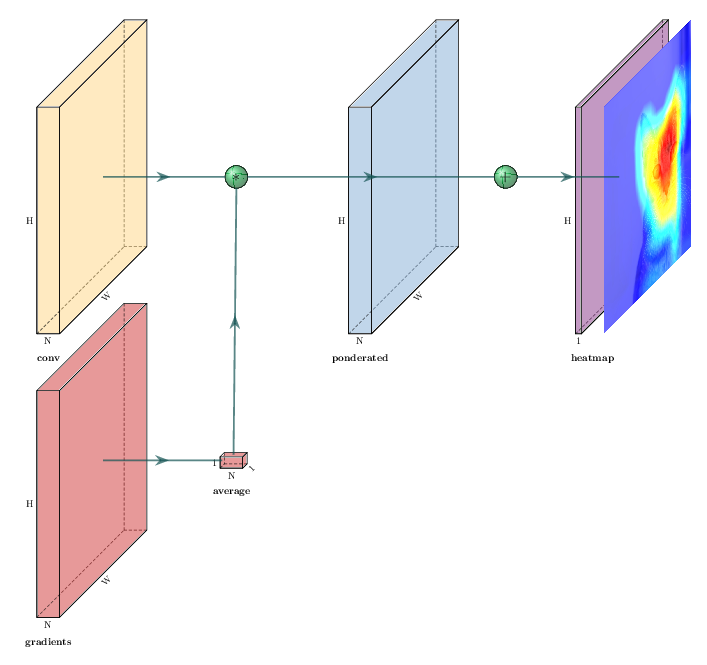The implementation follows this idea and does not differ much from the previous algorithms presented.

``````import cv2
import numpy as np
import tensorflow as tf

IMAGE_PATH = './cat.jpg'
LAYER_NAME = 'block5_conv3'
CAT_CLASS_INDEX = 281

img = tf.keras.preprocessing.image.img_to_array(img)

model = tf.keras.applications.vgg16.VGG16(weights='imagenet', include_top=True)

# Create a graph that outputs target convolution and output

# Get the score for target class
loss = predictions[:, CAT_CLASS_INDEX]

output = conv_outputs

# Build a ponderated map of filters according to gradients importance
cam = np.ones(output.shape[0:2], dtype=np.float32)

for index, w in enumerate(weights):
cam += w * output[:, :, index]

# Heatmap visualization
cam = cv2.resize(cam.numpy(), (224, 224))
cam = np.maximum(cam, 0)
heatmap = (cam - cam.min()) / (cam.max() - cam.min())

cam = cv2.applyColorMap(np.uint8(255*heatmap), cv2.COLORMAP_JET)

output_image = cv2.addWeighted(cv2.cvtColor(img.astype('uint8'), cv2.COLOR_RGB2BGR), 0.5, cam, 1, 0)
``````

However, a subtlety used in the Grad CAM paper is called Guided Backpropagation.It consists in eliminating elements that acts negatively towards the decision, by zeroing-out the negative gradients or gradients associated with a negative value of the filter.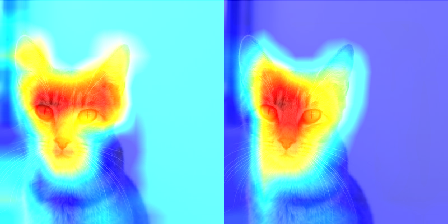(left) Grad CAM, (right) Grad CAM + Guided Backpropagation

Tensorflow offers the `tf.RegisterGradient` method to define a new gradient method, which combined with the `gradient_override_map` helps switch the behavior for our ReLU layers.

``````@tf.RegisterGradient("GuidedRelu")
gate_f = tf.cast(op.outputs > 0, "float32")  # Filter must be activated
return gate_f * gate_R * grad

with tf.Graph().as_default() as g:
model = tf.keras.applications.resnet50.ResNet50(weights='imagenet', include_top=True)

# Do stuff here
``````

Unfortunately, if you try to run this operation, Tensorflow informs you that `tf.cast` is no longer supported in version `2.0:tf.GradientTape.gradients() does not support graph control flow operations like tf.cond or tf.while at this time.`

As we use the guide only at inference time, we can perform this operation after gradients computation rather than during. It implies a small change after the `GradientTape` call.

``````with tf.GradientTape() as tape:
loss = predictions[:, CAT_CLASS_INDEX]

output = conv_outputs

# Apply guided backpropagation
gate_f = tf.cast(output > 0, 'float32')
gate_r = tf.cast(grads > 0, 'float32')
``````

guided_backprop.py

Full gist for the Grad CAM implementation (with guided backpropagation) is available here.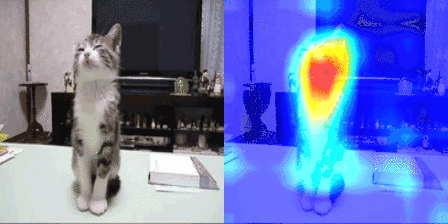Those methods are all implemented in tf-explain, which you can use on your trained models or in Keras callbacks. I’ll write more articles with additional methods as we integrate them into tf-explain, follow me on Twitter to get notified!

## What is TensorFlow? TensorFlow

An end-to-end open-source platform for Machine Learning. Before we start with TensorFlow, we will need to know what machine learning and deep learning technologies are.

## Serving TensorFlow models with TensorFlow Serving

This is a detailed guide on how to create TensorFlow models and then deploy them using TensorFlow Serving

## A TensorFlow Modeling Pipeline using TensorFlow Datasets and TensorBoard

This article investigates TensorFlow components for building a toolset to make modeling evaluation more efficient. Specifically, TensorFlow Datasets (TFDS) and TensorBoard (TB) can be quite helpful in this task.

## Keras vs. Tensorflow - Difference Between Tensorflow and Keras

Keras vs Tensorflow - Learn the differences between Keras and Tensorflow on basis of Ease to use, Fast development,Functionality,flexibility,Performance etc

## Deployment of a TensorFlow model to Production using TensorFlow Serving

Deploy a Deep Learning Model to Production using TensorFlow Serving.